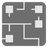Net Game
Simple
English RU

This mission is an adaptation of the "Net" game (from Simon Tatham's Portable Puzzle Collection). If you are lost or just want to play, the game is available here.

You have a rectangular grid with tiles you can only rotate, but it's a bit messy: tiles aren’t connected like they are supposed to be. You’ll have to put them in order by rotating each tile to reassemble the network.

The successful solution will be an entirely connected network with no closed loops.

There are four types of tiles:

• Dead ends• Straight roads• 90° turns• T junctionsTiles will be schematized by the directions to which they point (North, West, South, East), and more precisely with a string of directions (for example 'NE' if the tile is pointing to the Northeast, then it's a 90° turn).

Note that the order of directions in the string is meaningless (for example: 'NSE' and 'ENS' are the same) for input and output.

```[['NW' , 'S'  , 'N'  , 'E'  , 'SE'],       [['SE' , 'W'  , 'S'  , 'E'  , 'SW'],
['NS' , 'W'  , 'NWE', 'NWE', 'SE'],        ['NS' , 'S'  , 'NSE', 'WSE', 'NW'],
['WSE', 'NSE', 'NWE', 'W'  , 'E' ],  ===\  ['NSE', 'NWE', 'NWS', 'N'  , 'S' ],
['WE' , 'WS' , 'WSE', 'SE' , 'WE'],  ===/  ['NS' , 'SE' , 'NWE', 'SW' , 'NS'],
['W'  , 'NE' , 'N'  , 'NW' , 'WS']]        ['N'  , 'NE' , 'W'  , 'NE' , 'NW']]
```

Input: A list of lists of strings.

Output: A list/tuple of lists/tuples of strings.

Example:

```checkio([['NW' , 'S'  , 'N'  , 'E'  , 'SE'],
['NS' , 'W'  , 'NWE', 'NWE', 'SE'],
['WSE', 'NSE', 'NWE', 'W'  , 'E' ],
['WE' , 'WS' , 'WSE', 'SE' , 'WE'],
['W'  , 'NE' , 'N'  , 'NW' , 'WS']]) == [['SE' , 'W'  , 'S'  , 'E'  , 'SW'],
['NS' , 'S'  , 'NSE', 'WSE', 'NW'],
['NSE', 'NWE', 'NWS', 'N'  , 'S' ],
['NS' , 'SE' , 'NWE', 'SW' , 'NS'],
['N'  , 'NE' , 'W'  , 'NE' , 'NW']]
```

To play the puzzles / tests yourself: 1 2 3 4 5 6 7 8 9 10 11 12

Preconditions:

• Given puzzles are solvable.
• 3 ≤ len(grid) ≤ 25, 3 ≤ len(grid) ≤ 40.
• all(len(row) == len(grid) for row in grid).